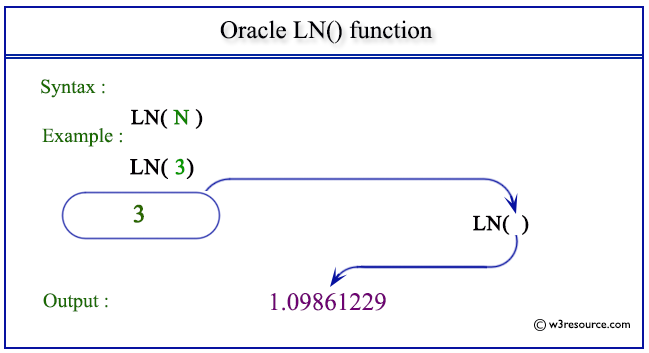# Oracle LN() function

## Description

Oracle LN() returns the natural logarithm of a number that is the base e logarithm of the number, where the number is greater than 0.
The function takes any numeric or nonnumeric data type (can be implicitly converted to a numeric data type) as an argument.
If the argument is BINARY_FLOAT, then the function returns BINARY_DOUBLE. Otherwise the function returns the same numeric data type as the argument.

Syntax:

`LN (N)`

Where N is a number.

Pictorial Presentation of LN() functionExample:

The statement below returns the natural logarithm of 3.

``````SELECT LN(3) FROM dual;
```
```

Here is the result.

```     LN(3)
----------
1.09861229
```

Previous: FLOOR
Next: LOG

﻿